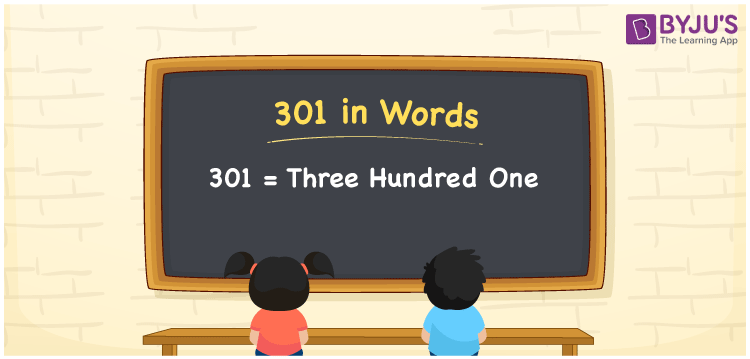# 301 in Words

301 in words is written as Three Hundred One. In both the International System of Numerals and the Indian System of Numerals, 301 is written as Three Hundred One. The number 301 is a Cardinal Number as it describes some quantity. For example, “There are 301 pages in that book”.

 301 in Words Three Hundred One Three Hundred One in Number 301

## 301 in English Words

We write 301 in English Words using the letters of the English alphabet. Therefore, we read 301 in English as “Three Hundred One.”## How to Write 301 in Words?

To write 301 in words, we shall use the place value chart. In the place value chart, write 1 in the ones, 0 in the tens, and 3 in the hundreds. Now let us make a place value chart to write the number 301 in words.

 Hundreds Tens Ones 3 0 1

Thus, we can write the expanded form as

3 × Hundred + 0 × Ten + 1 × One

= 3 × 100 + 0 × 10 + 1 × 1

= 300 + 0 + 1

= 301

= Three Hundred One.

301 is a natural number, the successor of 300 and the predecessor of 302.

301 in words – Three Hundred One

• Is 301 an odd number? – Yes
• Is 301 an even number? – No
• Is 301 a perfect square number? – No
• Is 301 a perfect cube number? – No
• Is 301 a prime number? – No
• Is 301 a composite number? – Yes

## Frequently Asked Questions on 301 in Words

### How to write 301 in words?

301 in words is written as Three Hundred One.

### How to write 301 in words in the International and Indian System of Numerals?

In both the systems of numerals, 301 is written as Three Hundred One.

### How to write 301 in a place value chart?

In the place value chart, write 1 in ones, 0 in tens, and then 3 in the hundreds place.